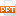# Chapter 5 Inverse Functions - Section 5.8: Indeterminate forms and l’hospital’s rule

We can evaluate this last limit either by using l’Hospital’s Rule a third time or by writing tan x as (sin x)/(cos x) and making use of our knowledge of trigonometric limits.26 trang | Chia sẻ: vutrong32 | Ngày: 19/10/2018 | Lượt xem: 308 | Lượt tải: 0
Bạn đang xem trước 20 trang tài liệu Chapter 5 Inverse Functions - Section 5.8: Indeterminate forms and l’hospital’s rule, để xem tài liệu hoàn chỉnh bạn click vào nút DOWNLOAD ở trên
SECTION 5.8INDETERMINATE FORMS AND L’HOSPITAL’S RULEP*5.8INDETERMINATE FORMSSuppose we are trying to analyze the behavior of the functionAlthough F is not defined when x = 1, we need to know how F behaves near 1. In particular, we would like to know the value of the limitP*5.8INDETERMINATE FORMSIn computing this limit, we can’t apply Law 5 of limits (Section 1.4) because the limit of the denominator is 0. In fact, although the limit in Expression 1 exists, its value is not obvious because both numerator and denominator approach 0 and is not defined.P*5.8INDETERMINATE FORM —TYPE 0/0In general, if we have a limit of the formwhere both f(x) → 0 and g(x) → 0 as x → a, then this limit may or may not exist.It is called an indeterminate form of type .We met some limits of this type in Chapter 1. P*5.8INDETERMINATE FORMSFor rational functions, we can cancel common factors:We used a geometric argument to show that:P*5.8INDETERMINATE FORMSHowever, these methods do not work for limits such as Expression 1.Hence, in this section, we introduce a systematic method, known as l’Hospital’s Rule, for the evaluation of indeterminate forms. Another situation in which a limit is not obvious occurs when we look for a horizontal asymptote of F and need to evaluate the limitP*5.8INDETERMINATE FORMSIt isn’t obvious how to evaluate this limit because both numerator and denominator become large as x → ∞.There is a struggle between the two. If the numerator wins, the limit will be ∞. If the denominator wins, the answer will be 0. Alternatively, there may be some compromise—the answer may be some finite positive number. P*5.8INDETERMINATE FORM —TYPE ∞/∞ In general, if we have a limit of the form where both f(x) → ∞ (or – ∞) and g(x) → ∞ (or – ∞), then the limit may or may not exist.It is called an indeterminate form of type ∞/∞.P*5.8INDETERMINATE FORMSWe saw in Section 1.6 that this type of limit can be evaluated for certain functions—including rational functions—by dividing the numerator and denominator by the highest power of x that occurs in the denominator.For instance,P*5.8INDETERMINATE FORMSThis method, though, does not work for limits such as Expression 2. However, L’Hospital’s Rule also applies to this type of indeterminate form.P*5.8L’HOSPITAL’S RULESuppose f and g are differentiable and g’(x) ≠ 0 on an open interval I that contains a (except possibly at a). Suppose or that In other words, we have an indeterminate form of type or ∞/∞.Then, if the limit on the right exists (or is ∞ or – ∞).P*5.8NOTE 1L’Hospital’s Rule says that the limit of a quotient of functions is equal to the limit of the quotient of their derivatives—provided that the given conditions are satisfied. It is especially important to verify the conditions regarding the limits of f and g before using the rule. P*5.8NOTE 2The rule is also valid for one-sided limits and for limits at infinity or negative infinity.That is, ‘‘x → a’’ can be replaced by any of the symbols x → a+, x → a–, x →∞, or x → – ∞. P*5.8NOTE 3For the special case in which f(a) = g(a) = 0, f ’ and g’ are continuous, and g’(a) ≠ 0, it is easy to see why the rule is true.P*5.8NOTE 3In fact, using the alternative form of the definition of a derivative, we have: The general version of l’Hospital’s Rule is more difficult; its proof can be found inAppendix B.P*5.8Example 1FindSOLUTION Thus, we can apply l’Hospital’s Rule: P*5.8Example 2CalculateSOLUTIONWe haveSo, l’Hospital’s Rule gives:P*5.8Example 2 SOLUTIONAs ex → ∞ and 2x → ∞ as x → ∞, the limit on the right side is also indeterminate.However, a second application of l’Hospital’s Rule gives: P*5.8Example 3CalculateSOLUTIONAs ln x → ∞ and as x → ∞, l’Hospital’s Rule applies:Notice that the limit on the right side is now indeterminate of type .P*5.8Example 3 SOLUTIONHowever, instead of applying the rule a second time as we did in Example 2, we simplify the expression and see that a second application is unnecessary: P*5.8Example 4FindSOLUTIONNoting that both tan x – x → 0 and x3 → 0 as x → 0, we use l’Hospital’s Rule:P*5.8Example 4 SOLUTIONAs the limit on the right side is still indeterminate of type , we apply the rule again:Since , we simplify the calculation by writing:P*5.8Example 4 SOLUTIONWe can evaluate this last limit either by using l’Hospital’s Rule a third time or by writing tan x as (sin x)/(cos x) and making use of our knowledge of trigonometric limits. P*5.8Example 4 SOLUTIONPutting together all the steps, we get:P*5.8Example 5FindSOLUTIONIf we blindly attempted to use l-Hospital’s rule, we would get:P*5.8Example 5 SOLUTIONThis is wrong. Although the numerator sin x → 0 as x → p –, notice that the denominator (1 – cos x) does not approach 0.So, the rule can’t be applied here.The required limit is, in fact, easy to find because the function is continuous at p and the denominator is nonzero there:

Các file đính kèm theo tài liệu này:

•indeterminate_forms_4851.ppt
Tài liệu liên quan